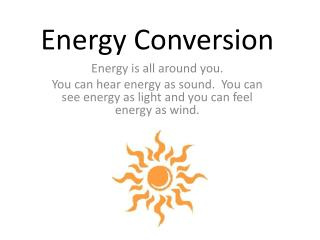DownloadDownload PresentationEnergy Conversion

# Energy Conversion

Télécharger la présentation## Energy Conversion

- - - - - - - - - - - - - - - - - - - - - - - - - - - E N D - - - - - - - - - - - - - - - - - - - - - - - - - - -
##### Presentation Transcript

1. Energy Conversion Energy is all around you. You can hear energy as sound. You can see energy as light and you can feel energy as wind.

2. What is Electricity? Electricity is the transfer or energy in the form of negative charges. It is important to understand that we do not MAKE electricity. We convert other forms of energy into electricity (kWh).

3. Basic Electricity Concepts Voltage : Potential to Move Charge (volts) Current : amount of charge moving past a given point each second(amperes) Resistance: tendency of a material to oppose the flow of electricity (ohms) Electrical Power:the rate at which electrical energy is transferred by an electric circuit (kW)

4. Ohm’s Law Voltage = Current x Resistance V=IR Power = Current x Voltage P=IV

5. What causes electricity to flow? • Electrons in a circuit have potential energy. • The difference between two different areas of electrical potential energy is called potential difference or voltage. • A voltage source (battery or generator) creates a potential difference. • Electricity flows from high voltage to lower voltage.

6. Battery A device that converts chemical energy into electrical energy.

7. Transformers A transformer is a device that increases or decreases the voltage. A current passes through the primary coil. The iron core becomes an electromagnet. This changing magnetic field induces a current in the secondary coil.

8. Step-Up Transformer A step-up transformer increases voltage. The secondary coil has more turns than the primary coil.

9. Step-Down Transformer A step-down transformer decreases voltage. The primary coil has more turns than the secondary coil.

10. The Faraday Effect Copper Wire Magnets Motion Electricity

11. From Mechanical to Electrical Energy Moving charges like those in an electric current, produce magnetic fields and moving magnetic fields create electric current.

12. Electric Generators G A generator produces electric current by rotating a coil of wire in a magnetic field. This process is called electromagnetic induction. Mechanical Energy Electrical Energy

13. Connection to Wind Energy Wind moves against the blades of a wind turbine. The energy forces the propeller to spin which subsequently spins a generator inside the turbine. As the generator is rotated, the magnets that are moving around an electrical wire inside the turbine causes electrons to move through the wire. Thus, electricity is created out of wind energy by the wind turbine.

14. Electric Motors M An electric motor is a device that changes electrical energy into mechanical energy. It contains an electromagnet that is free to rotate between the poles a permanent fixed magnet. The coil of the electromagnet if attached to an electrical source Electrical Energy Mechanical Energy

15. Renewable Energy • Resources that can be replaced in a relatively short period of time. • Most renewable energy sources originate either directly or indirectly from the sun. Ex. Hydroelectric, solar energy, geothermal energy, biomass, hydrogen fuel cell.

16. Nonrenewable Energy • Resources that exist in limited quantities and, once used, cannot be replaced except over the course of millions of years. Ex. Coal, oil natural gas, uranium